# Thermodynamic Expression of Economics

#### By Mark Ciotola

First published on June 15, 2019. Last updated on May 23, 2021.

# Thermodynamic Expression of Economics

### By Mark Ciotola

First published on June 15, 2019. Last updated on May 23, 2021.

This course describes economics concepts in terms of  thermodynamic principles.

This course describes economics concepts in terms of  thermodynamic principles.

1. ## 1 Introduction to a Thermodynamic Expression of Economics

First published on May 17, 2019. Last updated on May 23, 2021.

Thermodynamic Expression of Economics provides an exploration of how economic concepts can be expressed in terms of thermodynamic principles. People exist in a physical universe and are themselves comprised of physical matter. Both our  desired and transactions are ultimately expressed in a physical reality. Living organisms and systems are essentially thermodynamic processes. Thermodynamics is the branch of physics that involves the consumption of resources and their transformation into productivity.

What is economics?

“Economics is the social science that studies the choices that individuals, business, governments, and entire societies make as they cope with scarcity, and the incentives that influence and reconcile those choices.”

The term scarcity includes physical constraints such as limited resources. For example, many essential minerals such as copper and gold, are not freely available in unlimited quantities. Neither is water or clean air in many places in the world. Scarcity, in a sense, also involves physical constraints such as efficiency, which is often a matter of life and death for both living organisms and business ventures.

Unsurprisingly, it is possible to express many concepts in economics using thermodynamics. This has the benefit that economic models can be constrained using physics, and physics can provide a deeper understanding of economics.

Thermodynamics, Energy And Thermodynamics, Course.Cafe

## Reference:

1. Arik Levenson (citing Parkin), Economics 001 Principles of Microeconomics

2. ## 2 Supply and Demand

First published on April 30, 2021. Last updated on May 23, 2021.

Supply and Demand theory is at the heart of economics. If you understand supply and demand theory, you can begin talking like an economist. Add some thermodynamics to dazzle everyone. Supply and Demand is a good place to begin our discussion of economics.

3. ## 2.1 Fourier’s Law of Heat Conduction

First published on . Last updated on May 23, 2021.

Fourier’s Law of Conduction concerns thermal energy flowing from a warmer body of to a cooler body through a thermal conductor. We call the bodies reservoirs. Flowing thermal energy is often called heat.

Let us consider a thermal conductor operating upon in exhaustible reservoirs. The hot reservoir could be a flame or a large tank of hot water. The cold reservoir could be radiator fin or cooling pond. The hot reservoir has thermal energy to spare, and the cold reservoir wants some of that energy.  The rate of thermal energy transfer is known as Q. The “hot” reservoir has a temperature of TH, and the “cold” reservoir has a temperature of TC. The conductor is of area (cross section) A, and of length L.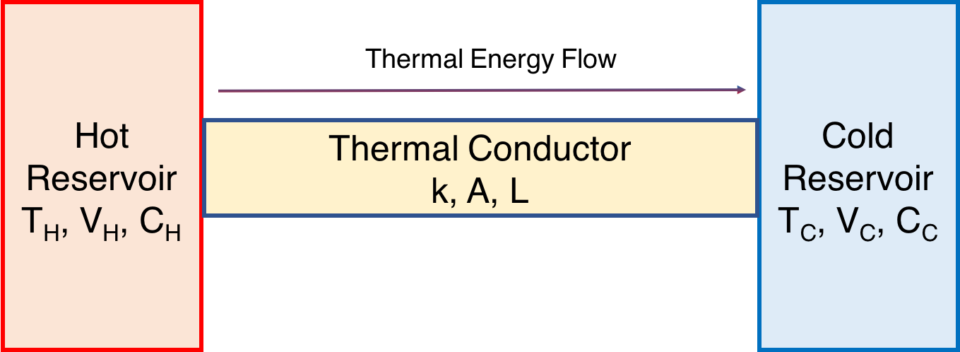If you are a knowledgeable engineer or scientist, you might point out that such thermal reservoirs have many important characteristics such as volume and specific heat capacity, but here we will simplify matters and assume that those are identical for both reservoirs and that there are no phase changes. Only their temperatures differ, the hot reservoir being at 300 and 500 “degrees” Kelvin (actually a unit just called Kelvin (K) with is similar to degrees). So the temperature difference is 200 K.

The flow of thermal energy is:

$$\Large{\frac{dQ}{dt}}=kA\frac{T_H – T_C}{L}$$.

The quantity of heat energy lost by the warmer body is identical to the quantity of heat energy gained by the cooler body. This must be true according to the First Law of Thermodynamics in order for energy to be conserved. That is, energy neither gained nor lost for the overall system. (Our conductor is well insulated except where it contacts the reservoirs).

Notice how the higher the temperature difference, the greater will be the flow of thermal energy. A large difference shall result in a large flow. A small difference will result in a small flow.

Note: Some thermodynamics textbooks or chapters begin with a discussion of Newton’s Law of Cooling. Fourier’s Law is a broader principle that encompasses Newton’s Law of Cooling.

4. ## 2.2 Supply and Demand

First published on May 17, 2019. Last updated on May 12, 2021.

## Introduction

Supply and Demand theory models at which prices consumers will purchase a product, at what prices will producers sell a product, and the resulting market price for the product. Supply and demand provides a framework for understanding pricing and sales volume. Although real life pricing sometimes deviates from this idealized theory, the Supply and Demand approach can help identify why those deviations exist.

## Supply Function

The supply function is the price at which producers will sell a product as a function of quantity sold. According to theory, producers will demand a higher price for each additional unit of product sold, due to increasing marginal costs. Let’s use gold as an example. Each additional unit of gold mined requires gold producers to mine more deeply into the ground (or use lower grade ore), which is more expensive. In summary, suppliers tend to charge more money per additional unit supplied, at least in the short run.

## Demand Function

The demand function is the price at which consumers will buy a product as a function of quantity purchased. According to theory, consumers will only pay a lower price for each additional unit of product purchased, due to a decreasing marginal utility. (Utility means the value the the product to consumers). Continuing with gold for an example, consumers only really need a small amount of  gold. They might be willing to pay all their savings for gold engagement jewelry. They might still be willing to pay a lot of money per gram for additional gold jewelry. Beyond that, most people do not have a high need or strong desire for more gold, and would not be willing to pay as much for more of it.

## Determining the Market Price

These functions can be plotted, with the axes being price and quantity. Where these two functions intersect indicates both market price and quantity produced. The plots shown are examples. Actual plots may vary.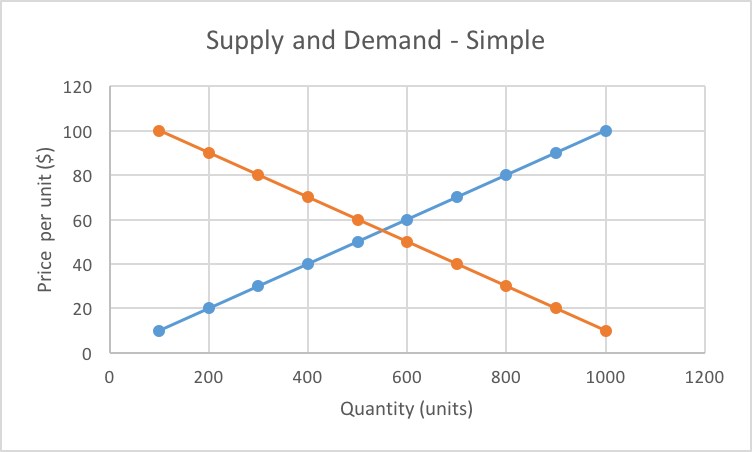Market price and quantity are where supply and demand functions meet to determine market price.

The rising plot represents the Supply Function. The falling plot represents and Demand function. Where they meet is the price at which the supplier (seller) and “demander” (buyer or customer) agree to conduct the transaction. Where they meet also shows the quantity of goods or services transferred.

5. ## 2.3 Expressing Supply and Demand Thermodynamically

First published on . Last updated on May 12, 2021.

## Recall Fourier’s Law of Conduction

Recall the example of a thermal conductor operating upon exhaustible, nonrenewable reservoirs.This is analogous to bridging market supply with demand. The hot reservoir has thermal energy to spare, and the cold reservoir wants some of that energy. So then, the hot reservoir has a supply function and the cold reservoir has a demand function.

The cold reservoir really “wants” that first bit of thermal energy a lot due the the high temperature difference! So it is willing to pay a high “price” for it. However, it wants each next bit of energy less and less, as its thermodynamic “hunger” becomes satisfied. Hence, demand slopes downward, just as the temperature difference does.

Eventually the two temperatures become equal, which is analogous to having satisfied all of the customer demand. This is shown in the thermodynamic supply and demand plot shown below.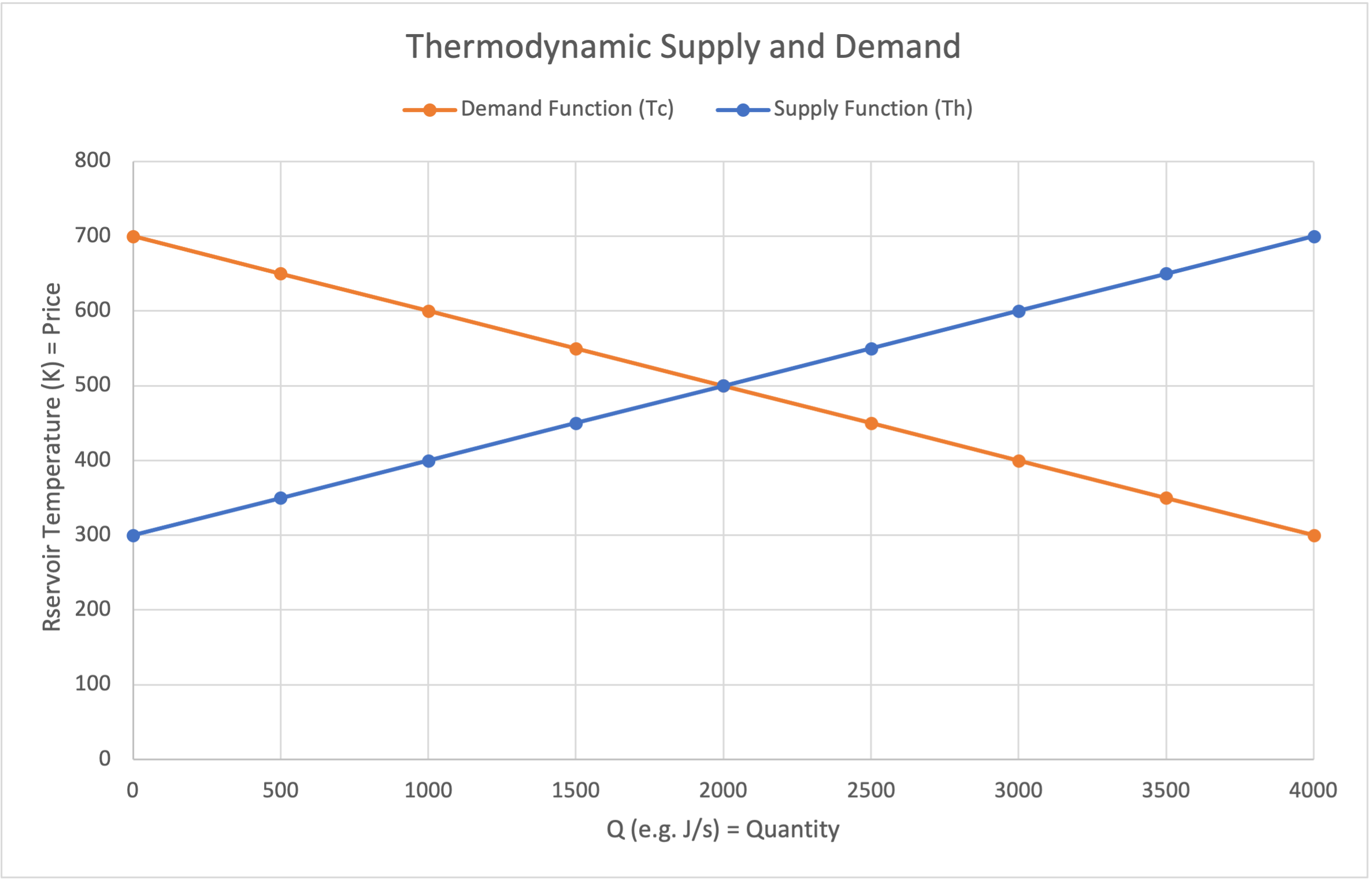Thermodynamic expression of supply and demand

Per Fourier’s Law, the flow of thermal energy is shown at the intersection of the function plots. The temperature of the intersection will be that of an intermediary point on the conductor.

## Market Price Equilibrium

Where there are both continuous sources of supply and demand, the market will eventually reach a market equilibrium where these two curves meet. Since such requires a continual flow of goods, this can be said to have reached dynamic equilibrium.

### Sales Volume

Even at equilibrium, what is the sales volume? From where the supply and demand curves meet, we know the quantity transferred. For example, if the heat capacity of the hot reservoir is 1, and the temperature difference is 200 K, then there are 200 J of heat that can be spread around. Since these are, limited exhaustible reservoirs, their temperature increases when they receive heat and it decreases when they lose heat. Since the reservoir have an equal heat capacity, transferring 100 J of thermal energy will reduce the hot reservoir temperature by 100K and increase the cold reservoir temperature by 100 J, in which case they shall be of equal temperature (400K) and their “hot” hot “cold” labels will have become meaningless. This thermodynamic equilibrium is analogous to market equilibrium.

Note that the equilibrium price and quantity will be different for different reservoir characteristics, just as different suppliers and customers each how their own differing characteristics. Also, if the reservoirs are inexhaustible so that their temperature never changes, heat will flow, and their is a equilibrium quantity (called dynamic equilibrium), but the equilibrium temperature would have a different meaning in this context.

6. ## 3 Microeconomics

First published on April 30, 2021. Last updated on April 30, 2021.

Microeconomics concerns scarcity and decision-making at the level of the firm or enterprise. Ultimately, supply and demand governs all of the enterprises in a market as a group.  Microeconomics drills deeper, to see what is going on for the individual firm. There can be a great deal of variability among firms, even in the same industry, so this is important analysis both at the firm level, at even at the market level to gain a full understanding.

7. ## 3.1 Heat Engines And Production

First published on . Last updated on May 12, 2021.

### Heat Engines and Work

Instead of bridging a hot and a cold reservoir with a simple conductor, we can instead place a heat engine in between them.

A heat engine is a device that can use the flow of thermal energy to perform work. Such work can many things such include pumping water, compressing a spring, or running and electric generator. Below we see a hypothetical heat engine turning a wheel, such as to run a generator.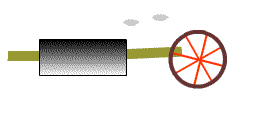A heat engine will typically operate between  hot and a cold reservoir as shown below. The hot reservoir is shown on the left and the cold reservoir is shown on the right.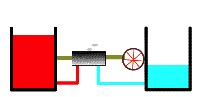High temperature thermal energy will flow into the heat engine. A lesser amount of thermal energy will flow out of the hear reservoir.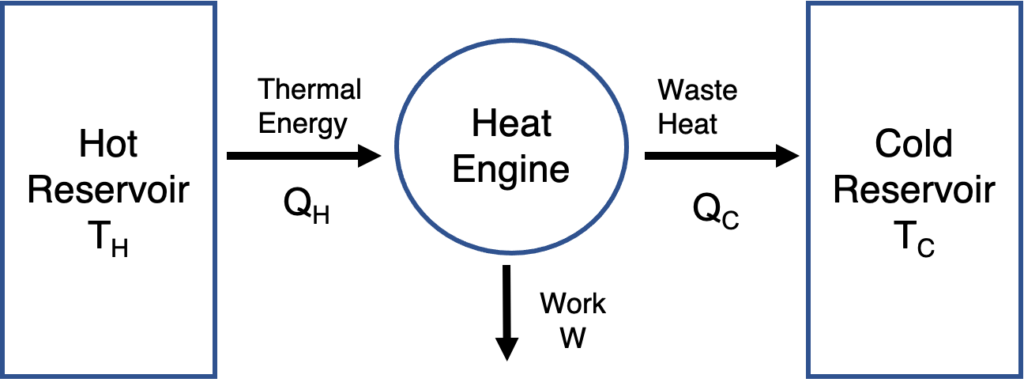Work and energy flow through a thermal conductor

The difference between thermal energy into and out of the heat engine is called work.

$$Q_{in} = Work + Q_{out}$$

### Work and Productivity

Work is a form of physical productivity that may represent economic productivity as well. Physical work can used to produce electricity, manufactured goods and provide many services. Work is the useful products of physical and economic processes.

$$Production = Work$$.

8. ## 3.2 Costs, Revenues and Profits

First published on May 17, 2019. Last updated on May 12, 2021.

## Introduction

Microeconomics concerns individual and firm-level decisions regarding the allocation of resources. There are several important concepts to analyze a firm-level venture. We will just consider a single product firm for the sake of simplicity. At its most basic level, microeconomics considers at what volumes and prices it makes sense for a enterprise to continue producing a good or service. Hence it is quite important for decision-making.

## Revenues, Costs and Profits

### General Definitions

Revenue (R) is the amount of money a firm receives in payment for a product sold.

Costs (C) are how much money a firm pays to make a product.

Profit (P) is the amount left over when cost is subtracted from revenue:

$$P = R – C$$

For example, a pizza requires $5 of ingredients, labor and overhead to make. A pizzeria can cell that pizza for$12. The profit would then be $7: $$7 = 12 – 5$$. The terms cost, revenue and profit are general terms, in that they can refer to one unit, lots of units or all units of product or service. For more realistic economic analysis we will need to be more specific. ### Definitions for Totals We will start by considering total cost, revenue and profit. Total Revenue (TR) is the sum of revenues a firm receives in payment for all units of the product sold. Total Cost (TC) is the sum of costs a firm pays to make all units of the product sold. Total Profit (TP) is the sum of profits obtained from all goods sold: $$TP = TR – TC$$. The pizzeria sells 100 pizzas each for$12, resulting in $1,200 in total revenue. The pizzas cost$5 to make, resulting in total costs of $500. Then the total profit would be$700:

$$700 = 1,200 – 500$$.

9. ## 3.3 Expressing Cost, Revenue and Profit Thermodynamically

First published on . Last updated on May 12, 2021.

Let’s start thinking about a heat engine and work in economic terms.  Let’s imagine a firm or venture is analogous to a heat engine operating across a hot and a cold reservoir. Thermal energy removed from the hot reservoir $$Q_H$$ is analogous to revenue (R). Thermal energy exhausted into the cold reservoir $$Q_C$$ is analogous to cost (C). Work is analogous to profit. This analogy is shown below.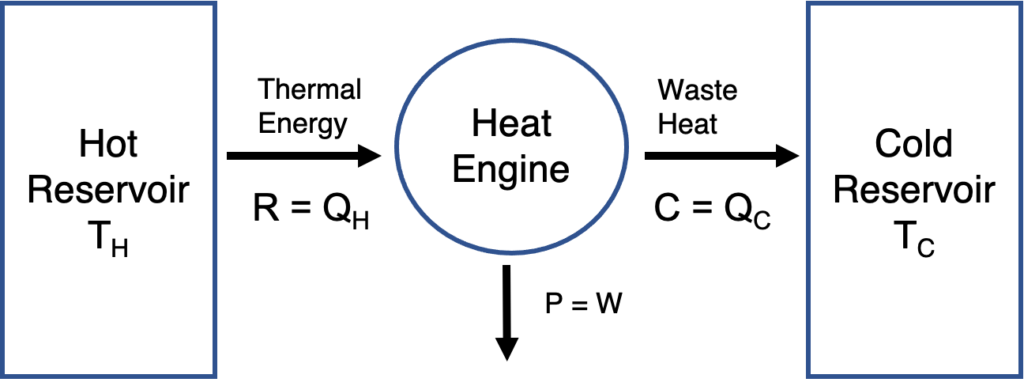A venture modeled as a heat engine

### Profits As Work

For example, you buy and sell widgets. You receive cash (revenue) when you sell widgets and you pay cash (costs) when you sell widgets. Here, there are no overhead costs. It’s a simple business.

Then,  Profit P would be:

$$W = Q_H – Q_C$$, or,

$$P = Q_H – Q_C$$, or,

$$P = R – C = Q_H – Q_c$$.

### Gross Margin

Let’s make our analogy more realistic and interesting. Continuing to model our firm a heat engine operating across a thermal difference, let’s examine gross profit margins. In business, the Gross Profit Margin, or simply Gross Margin (MG), can be expressed as:

$$GM = \frac{R – C}{R}$$

The form of this equation may look familiar. It is identical in form to the expression for Carnot efficiency:

$$\epsilon = \frac{T_H – T_C}{T_C} =1 – \frac{T_C}{T_H}$$

So we can now express Gross Margin thermodynamically:

$$GM = \epsilon = 1 – \frac{T_C}{T_H}$$

We can now express Marginal Profit thermodynamically:

$$P =Q_H~\epsilon = Q_H~(1 -\frac{T_C}{T_H})$$

In Summary, the work performed by the heat engine represents profit (P):

$$W = Q_H (1 – \frac{T_C}{ T_H})$$

Or combining expressions:

In Summary, the work performed by the heat engine represents profit (P):

$$GM = W =R (1 – \frac{C}{ R}) = Q_H (1 – \frac{T_C}{ T_H})$$

10. ## 3.4 Marginal Revenue, Cost and Profit

First published on April 30, 2021. Last updated on May 12, 2021.

### Definitions for Marginal Quantities

However, not all units of a product cost the same to make, nor are they always sold at the same price. So it is often of interest to determine the revenue, cost and profit of each additional unit produced and sold.

Marginal Revenue (MR) is the revenue received for one additional unit of product sold.

Marginal Cost (MC) is the cost paid to produce for one additional unit of product.

Marginal Profit (MP) is the profit obtained when producing one additional unit of product:

$$MP = MR – MC$$.

### Margins

Business people often talk of Gross Margin (GM), which is:

$$GM = \frac{Revenue – Cost~of~Goods~Sold}{Revenue}$$.

Here, the Cost of Goods Sold (COGS) is simply Costs, or C. So Gross Margin becomes:

$$GM = \frac{R – C}{R}$$.

### Fixed Costs

Fixed Costs (FC) are the minimum costs required to produce any amounts of units (even zero amount). For example, just to get into business, a pizzeria has to buy an oven.

Fixed costs can affect marginal costs. For example, if a pizza oven costs $1,000, and other costs are$5 per the above example, then the marginal cost for the first unit of pizza is $1,000 +$5 = $1,005. However, the marginal cost to make the second pizza would only be$5.

## Example

Let’s bring together several concepts now. Imagine you are a celebrity setting up an online retail firm selling tee shirts with your photo for $10. All orders are fulfilled using a third-party online service. They charge you$5 amount for each tee shirt.

Your marginal revenue is $10. Your marginal cost is$3. Your marginal profit is $7. If you sell 100 tee shirts, your total profit would be: $$Total~Profit = Total~Revenues – Total~Costs$$ $$400 = 100~shirts~ (\frac{7}{shirt} – \frac{3}{shirt})$$. What if you had to pay for an up-front set-up fee of$100 to process your photo? This would be a fixed cost, as well as a start-up cost. Even if you sold no tee shirts, you would still incur the $100 just to go into business. If you sold no shirts, you would have a$100 loss instead of a profit.

However, if you once again sold $100 shirts, your profit after fixed costs would be the$400 from above less the $100 start-up fee, for a net profit of$300.

What is the minimum amount of shirts you should expect to sell in order to decide to go into business? You will need to at least break-even, which means to cover the fixed costs plus expected marginal costs. Here, marginal profit will provide $4 per shirt, so it takes 25 shirts to cover the initial$100 investment.

## Other Important Quantities

There are other quantities that can affect a firm’s decisions.

Entry costs are the minimum cost to start a particular business. Such are closely related to fixed costs.

Opportunity Cost is the amount of profit given up by not pursuing a course of action. Typical sources of opportunity costs are risk and interest rates.

Risk is cost due to uncertainty.

Interest rates reflect a combination of costs due to risk and sacrificed opportunities. In reality, they can also represent bargaining power differentials.

The availability of Capital affects what courses of action are possible.

11. ## 4 Finance

First published on . Last updated on April 30, 2021.

While economics often concerns physical quantities, the abstraction of money is present for nearly all modern transactions. What is money, and how does it figure in our discussion of thermodynamics?

12. ## 4.1 The Nature of Money

First published on . Last updated on April 30, 2021.

In our model of supply and demand, goods and services flow from the supplier to the consumer (demander). This one way flow does not seem natural. In economics, money typically balances the flow.

In thermodynamics, a system maximizes its overall utility to maximizing its total entropy. Moving thermal energy from a hot to cold reservoir does so. Maximizing entropy is a way so saving evening things out.

In economics, we usually mean talk about maximizing economic utility, usually in terms of money. I a physical sense, this ultimately amount to the same thing, since utility is judged by humans, who are biochemical beings who ultimately themselves work to maximize overall entropy. Some scientists call this maximizing power instead of entropy, as in Maxwell’s Maximum Power Principle. (Even if this is not a conscious purpose, it is rather hardwired into people via evolution).